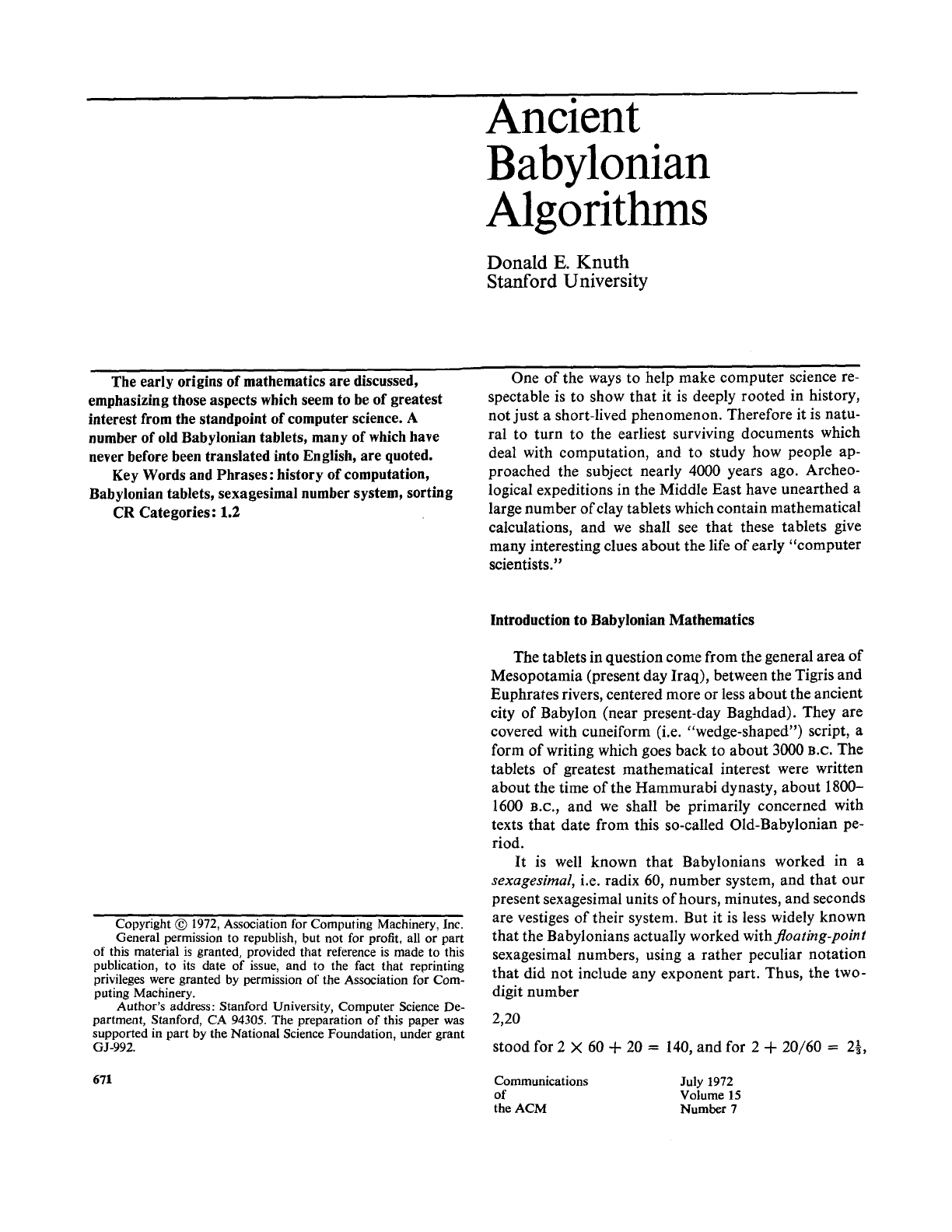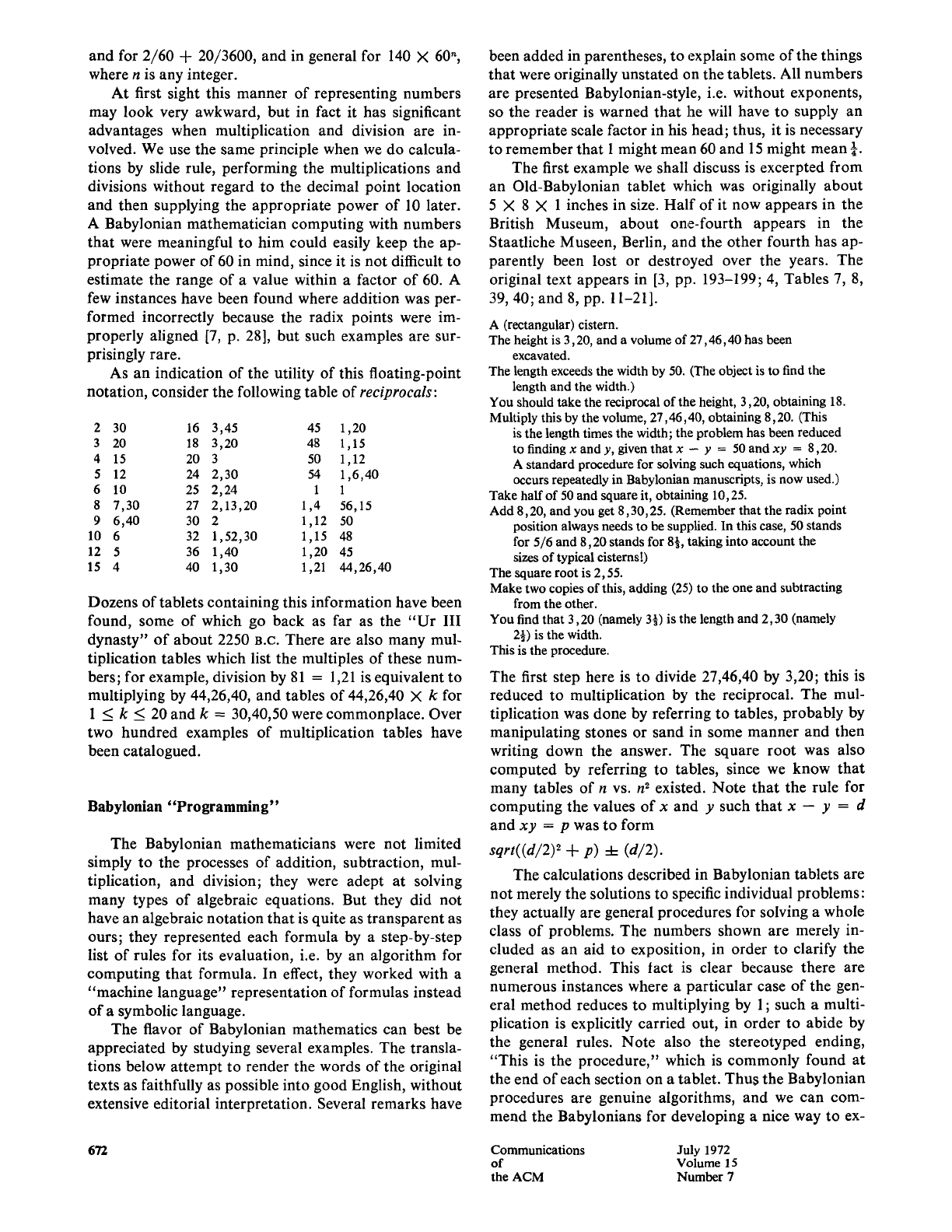### Cuneiform Cuneiform, originating around 3300 B.C., marked th...
In a sexagesimal system you use 60 as the base, unlike our modern b...
In other words, if you saw the two digit sexagesimal number 2,20 in...
Again remember that they inferred the exponent depending on the con...
In this context, a table of reciprocals is a lookup table that prov...
Here is an example of an actual Cuneiform tablet with a reciprocals...
This formula can be derived from the context of a quadratic equatio...
A cubit and a gar are units of measurement used in ancient Babyloni...
Linear interpolation is a method used to estimate values between tw...
The Plimpton tablet is a Babylonian clay tablet that dates back to ...Ancient
Babylonian
Algorithms
Donald E. Knuth
Stanford University
The early origins of mathematics are discussed,
emphasizing those aspects which seem to be of greatest
interest from the standpoint of computer science. A
number of old Babylonian tablets, many of which have
never before been translated into English, are quoted.
Key Words and Phrases: history of computation,
Babylonian tablets, sexagesimal number system, sorting
CR Categories: 1.2
One of the ways to help make computer science re-
spectable is to show that it is deeply rooted in history,
not just a short-lived phenomenon. Therefore it is natu-
ral to turn to the earliest surviving documents which
deal with computation, and to study how people ap-
proached the subject nearly 4000 years ago. Archeo-
logical expeditions in the Middle East have unearthed a
large number of clay tablets which contain mathematical
calculations, and we shall see that these tablets give
many interesting clues about the life of early "computer
scientists."
Copyright @ 1972, Association for Computing Machinery, Inc.
General permission to republish, but not for profit, all or part
of this material is granted, provided that reference is made to this
publication, to its date of issue, and to the fact that reprinting
privileges were granted by permission of the Association for Com-
puting Machinery.
Author's address: Stanford University, Computer Science De-
partment, Stanford, CA 94305. The preparation of this paper was
supported in part by the National Science Foundation, under grant
GJ-992.
671
Introduction to Babylonian Mathematics
The tablets in question come from the general area of
Mesopotamia (present day Iraq), between the Tigris and
Euphrates rivers, centered more or less about the ancient
city of Babylon (near present-day Baghdad). They are
covered with cuneiform (i.e. "wedge-shaped") script, a
form of writing which goes back to about 3000 B.C. The
tablets of greatest mathematical interest were written
1600 B.c., and we shall be primarily concerned with
texts that date from this so-called Old-Babylonian pe-
riod.
It is well known that Babylonians worked in a
sexagesirnal,
i.e. radix 60, number system, and that our
present sexagesimal units of hours, minutes, and seconds
are vestiges of their system. But it is less widely known
that the Babylonians actually worked
withfloating-point
sexagesimal numbers, using a rather peculiar notation
that did not include any exponent part. Thus, the two-
digit number
2,20
stood for 2 × 60 + 20 = 140, and for 2 + 20/60 = 2~,
Communications July 1972
of Volume 15
the ACM Number 7and for 2/60 + 20/3600, and in general for 140 X 60 ",
where n is any integer.
At first sight this manner of representing numbers
may look very awkward, but in fact it has significant
advantages when multiplication and division are in-
volved. We use the same principle when we do calcula-
tions by slide rule, performing the multiplications and
divisions without regard to the decimal point location
and then supplying the appropriate power of 10 later.
A Babylonian mathematician computing with numbers
that were meaningful to him could easily keep the ap-
propriate power of 60 in mind, since it is not difficult to
estimate the range of a value within a factor of 60. A
few instances have been found where addition was per-
formed incorrectly because the radix points were im-
properly aligned [7, p. 28], but such examples are sur-
prisingly rare.
As an indication of the utility of this floating-point
notation, consider the following table of
reciprocals:
2 30 16 3,45 45 1,20
3 20 18 3,20 48 1,15
4 15 20 3 50 1,12
5 12 24 2,30 54 1,6,40
6 10 25 2,24 1 1
8 7,30 27 2,13,20 1,4 56,15
9 6,40 30 2 1,12 50
10 6 32 1,52,30 1,15 48
12 5 36 1,40 1,20 45
15 4 40 1,30 1,21 44,26,40
Dozens of tablets containing this information have been
found, some of which go back as far as the
"Ur
III
dynasty" of about 2250 B.c. There are also many mul-
tiplication tables which list the multiples of these num-
bers; for example, division by 81 = 1,21 is equivalent to
multiplying by 44,26,40, and tables of 44,26,40 × k for
1 < k < 20 and k = 30,40,50 were commonplace. Over
two hundred examples of multiplication tables have
been catalogued.
Babylonian "Programming"
The Babylonian mathematicians were not limited
simply to the processes of addition, subtraction, mul-
tiplication, and division; they were adept at solving
many types of algebraic equations. But they did not
have an algebraic notation that is quite as transparent as
ours; they represented each formula by a step-by-step
list of rules for its evaluation, i.e. by an algorithm for
computing that formula. In effect, they worked with a
"machine language" representation of formulas instead
of a symbolic language.
The flavor of Babylonian mathematics can best be
appreciated by studying several examples. The transla-
tions below attempt to render the words of the original
texts as faithfully as possible into good English, without
extensive editorial interpretation. Several remarks have
been added in parentheses, to explain some of the things
that were originally unstated on the tablets. All numbers
are presented Babylonian-style, i.e. without exponents,
so the reader is warned that he will have to supply an
appropriate scale factor in his head; thus, it is necessary
to remember that 1 might mean 60 and 15 might mean ¼.
The first example we shall discuss is excerpted from
an Old-Babylonian tablet which was originally about
5 X 8 × 1 inches in size. Half of it now appears in the
British Museum, about one-fourth appears in the
Staatliche Museen, Berlin, and the other fourth has ap-
parently been lost or destroyed over the years. The
original text appears in [3, pp. 193-199; 4, Tables 7, 8,
39, 40; and 8, pp. 11-21].
A (rectangular) cistern.
The height is 3,20, and a volume of 27,46,40 has been
excavated.
The length exceeds the width by 50. (The object is to find the
length and the width.)
You should take the reciprocal of the height, 3,20, obtaining 18.
Multiply this by the volume, 27,46,40, obtaining 8,20. (This
is the length times the width; the problem has been reduced
to finding x and y, given that x -- y = 50 and
xy
= 8,20.
A standard procedure for solving such equations, which
occurs repeatedly in Babylonian manuscripts, is now used.)
Take half of 50 and square it, obtaining 10, 25.
Add 8,20, and you get 8,30, 25. (Remember that the radix point
position always needs to be supplied. In this case, 50 stands
for 5/6 and 8,20 stands for 8], taking into account the
sizes of typical cisterns!)
The square root is 2,55.
Make two copies of this, adding (25) to the one and subtracting
from the other.
You find that 3,20 (namely 3-~) is the length and 2,30 (namely
2½) is the width.
This is the procedure.
The first step here is to divide 27,46,40 by 3,20; this is
reduced to multiplication by the reciprocal. The mul-
tiplication was done by referring to tables, probably by
manipulating stones or sand in some manner and then
writing down the answer. The square root was also
computed by referring to tables, since we know that
many tables of n vs. n ~ existed. Note that the rule for
computing the values of x and y such that x -- y = d
and
xy = p
was to form
sqrt((d/2) ~ + p) 4-
(d/2).
The calculations described in Babylonian tablets are
not merely the solutions to specific individual problems:
they actually are general procedures for solving a whole
class of problems. The numbers shown are merely in-
cluded as an aid to exposition, in order to clarify the
general method. This tact is clear because there are
numerous instances where a particular case of the gen-
eral method reduces to multiplying by 1 ; such a multi-
plication is explicitly carried out, in order to abide by
the general rules. Note also the stereotyped ending,
"This is the procedure," which is commonly found at
the end of each section on a tablet. Thus the Babylonian
procedures are genuine algorithms, and we can com-
mend the Babylonians for developing a nice way to ex-
672
Communications July 1972
of
Volume 15
the
ACM Number 7